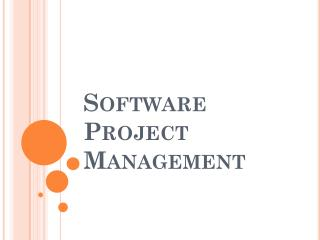DownloadDownload PresentationSoftware Project Management

Software Project Management

Download PresentationSoftware Project Management

- - - - - - - - - - - - - - - - - - - - - - - - - - - E N D - - - - - - - - - - - - - - - - - - - - - - - - - - -
Presentation Transcript

1. Software Project Management

2. Manager = Team + leadership • Software project management is the important task of planning, directing, motivating, and coordinating a group of professionals to accomplish software development • Project Visibility

3. Management Approaches • “process” v/s “project” • In process-oriented management, the management of the small tasks in the software life cycle is emphasized. • In project management, the team achieving the project is emphasized.

4. High-level process model for hierarchical team structure

5. Personal Software Process • The programmer spent 360 + 270 + 150 + 120 = 900 minutes to write and test a program of 160 LOC. • Assuming 5 hours per day (300 minutes/day), X spent effectively 3 days to program 160 LOC. • This gives a productivity of 53 LOC/day. When X’s manager schedules a week to code a 1000 LOC project, X is able to estimate that the project will take about 4 weeks.

6. Basic Measures • Budgeted Cost of Work (BCW):The estimated effort for each work task. • Budgeted Cost of Work Scheduled (BCWS):The sum of the estimated effort for each work task that was scheduled to be completed by the specified time. • Budget at Completion (BAC):The total of the BCWS and thus the estimate of the total effort for the project. • Planned Value (PV):The percentage of the total estimated effort that is assigned to a particular work task; PV = BCW/BAC. • Budgeted Cost of Work Performed (BCWP):The sum of the estimated efforts for the work tasks that have been completed by the specified time. • Actual Cost of Work Performed (ACWP): The sum of the actual efforts for the work tasks that have been completed.

7. Progress Indicators • Earned Value (EV) = BCWP/BAC = The sum of the PVs for all completed work tasks = PC = Percent complete • Schedule Performance Index(SPI) = BCWP / BCWS • Schedule Variance (SV) = BCWP - BCWS • Cost Performance Index(CPI) = BCWP / ACWP • Cost Variance (CV) = BCWP - ACWP

8. Example I • The BAC is the sum of the estimations. BAC = 330 days. BAC is an estimate of the total work. • On 4/1/01, tasks 1,2, and 4 have been completed. The BCWP is the sum of the BCWS for those tasks. So BCWP is 70 days. The earned value (EV) is 70/330, or 21.2 percent. • On 4/1/01 tasks 1 and 2 were scheduled to be completed and 1,2, and 4 were actually completed. So BCWP is 70 days and BCWS is 30 days. Thus, SPI is 70/30, or 233 percent. The SV = 70 days -30 days = +40 days, or 40 programmer-days ahead. • The ACWP is the sum of actual effort for tasks 1, 2, and 4. So, ACWP is 80 programmer-days. CPI is 70/80 = 87.5 percent. • The CV = 70 programmer-days -80 programmer-days = -10 programmer-days, or 10 programmer-days behind.

9. Example II • On 7/1/01, assume that task3 has also been completed using 140 days of actual effort, so BCWP is 190 and EV is 190/330, or 57.5 percent. • On 7/1/01, tasks 1, 2, 3, and 4 were actually completed. So BCWP is 190 days and BCWS is 250 days. Thus, SPI is 190/250 = 76 percent. • The SV is 190 programmer-days 250 programmer-days = 60 programmer-days, or 60 programmer days behind. • ACWP is the sum of actual effort for 1, 2, 3, and 4. So ACWP is 220 programmer-days. • Tasks 1 through 5 were scheduled to have been completed, but only 1 through 4 were actually completed. CPI is 190/220 = 86.3 percent, and CV is 190–220, or 30 programmer-days behind.

10. Example III • The project started on January 1 and should be finished by June 1. It is now March 1. Complete the following table. Calculate EV, SPI, SV, and CV. Determine whether the project is on time.

11. The project started on January 1 and should be finished by June 1. It is now March 1. Complete the following table. Calculate EV, SPI, SV, and CV. Determine whether the project is on time. BAC = 200 BCWS = 50 BCWP = 70 ACWP = 60 EV = 70/200 = 0.35 SV = 70 - 50 = 20 SPI = 70/50 = 1.4 CV= 70 - 60 = 10 The project is ahead of schedule.

12. Critical Practices I • Adopt continuous risk management. • Estimate cost and schedule empirically. • Use metrics to manage. • Track earned value. • Track defects against quality targets. • Treat people as the most important resource. • Adopt life cycle configuration management.1 • Manage and trace requirements.

13. Critical Practices II • Use system-based software design. • Ensure data and database interoperability. • Define and control interfaces. • Design twice, code once. • Assess reuse risks and costs. • Inspect requirements and design. • Manage testing as a continuous process. • Compile and smoke-test frequently.